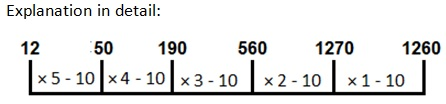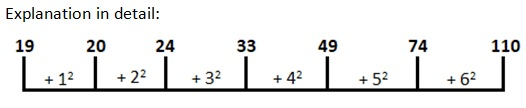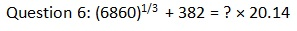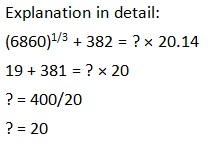# Quantitative Aptitude Quiz 2Quantitative Aptitude Quiz 2 consists of 10 new pattern questions asked in SBI PO, SBI Clerk, IBPS PO, IBPS Clerk exams. Candidates can practice such new pattern questions to get familiar with the actual type of questions asked in IBPS, SBI and other insurance exams:

Question 1: The difference between 3/5 of 7/5 of a number and 2/5 of 3/4 of the same number is 108. What is 7/20 of the number?

a. 95
b. 70
c. 100
d. 140
e. 35

b. 70
Explanation in detail:
Let the number be x.
(3x/5) × (7/5) – (2x/5) × (3/4) = 108
(21x/25) – (6x/20) = 108
27x/50 = 108
So, x = 200
Required answer = (7/20) × 200 = 70

Question 2: The sum of six consecutive numbers is 129. What is the average of the first, fifth and sixth number?

a. 19
b. 24
c. 22
d. 23
e. 20

c. 22
Explanation in detail:
Let the first number be x.
x + (x + 1) + (x + 2) + (x + 3) + (x + 4) + (x + 5) = 129
6x + 15 = 129
x = 19
Six consecutive numbers are 19, 20, 21, 22, 23 and 24.
Average of the first, fifth and sixth number.
= (19 + 23 + 24)/3
= 22

Question 3: Find out the wrong number in the following series:
12, 50, 190, 550, 1270, 1260

a. 50
b. 1270
c. 190
d. 550
e. 12

d. 550Question 4:Find out the wrong number in the following series:
19, 21, 24, 33, 49, 74, 110

a. 24
b. 49
c. 74
d. 33
e. 21

e. 21Question 5:If 19.99% of 650 is added to the product of 7 and 9, what is the approximate number obtained?

a. 143
b. 103
c. 193
d. 243
e. None of the above

c. 193
Explanation in detail:
19.99% is approximately equal to 20%
(20% of 650) + (7 × 9)
= (130 + 63)
= 193

Directions (6-10): What approximated value should come in place of the question mark (?) in the following questions? (Don’t calculate the exact value):a. 20
b. 30
c. 40
d. 10
e. 15

a. 20Question 7:44.896% of 7841 + 39.6% of 45 = ?

a. 3022
b. 3546
c. 5437
d. 3962
e. 4000

b. 3546
Explanation in detail:
? = 44.896% of 7841 + 39.6% of 45
? = 45% of 7840 + 40% of 45
? = 3528 + 18
? = 3546

Question 8: 63.88 ÷ 11.98 × 8.89 + 53 = ?

a. 150
b. 75
c. 85
d. 100
e. 175

d. 100
Explanation in detail:
? = 63.88 ÷ 11.98 × 8.89 + 53
? = 64 ÷ 12 × 9 + 53
? = 38 + 53
? = 100 (Approximately)

Question 9: 23.79 ÷ 5.99 + 35.87% of 499 = ?

a. 132
b. 184
c. 243
d. 341
e. 99

b. 184
Explanation in detail:
? = 23.79 ÷ 5.99 + 35.87% of 499
? = 24 ÷ 6 + 36% of 500
? = 4 + 180
? = 184

Question 10: 54.89% of 481.32 + 235 = 24.87 × ?

a. 20
b. 30
c. 39
d. 48
e. 10

a. 20
Explanation in detail:
54.89% of 481.32 + 235 = 24.87 × ?
55% of 480 + 235 = 25 × ?
264 + 235 = 25 × ?
? = 500/25
? = 20

IBPS Home

Back to Home